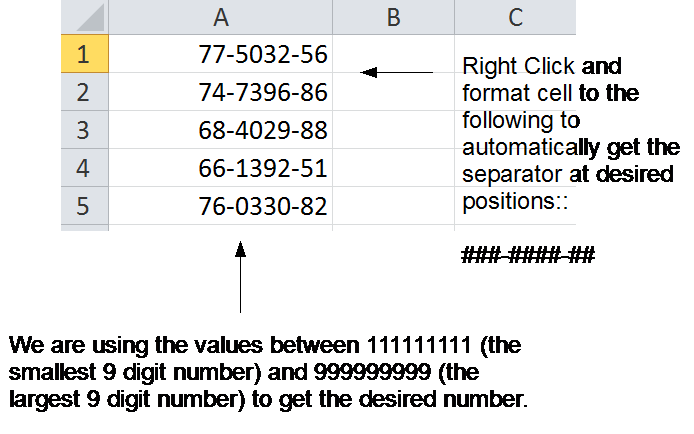# Generating Random Data In 5 Minutes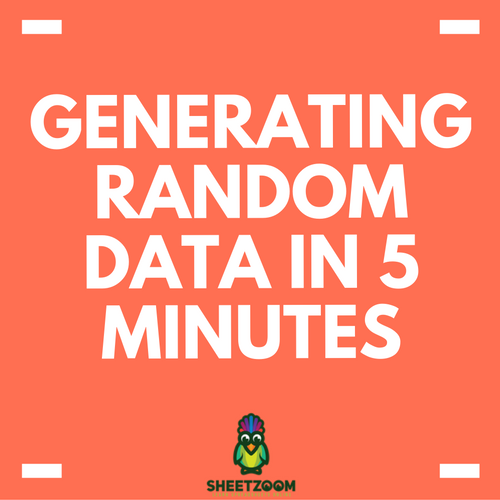When we are developing a model or trying to test some formula, we need data and when we don’t have one, we have to create it to suit our needs. This is frequently called dummy data. Toda we will show you how to create Dummy Data in 10 minutes so that you can test your formulas and models.

The MS Excel has a built-in function of RAND() and RANDBETWEEN() that serves the purpose. RAND() do not take any argument and returns a value between 0.00 and 1.00. On the other hand, RANDBETWEEN() can return data in a given range.

Generating Numerical Values:

Tip # 01:

If you need numbers between a given two given values use RANDBETWEEN(start_num,end_num)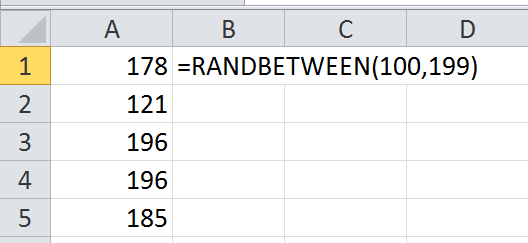Tip # 02:

If you need numbers between two given values that are less than 1 (i.e. decimal values) but use RANDBETWEEN() but divide the values by 100 i.e. RANDBETWEEN(start_num,end_num)/100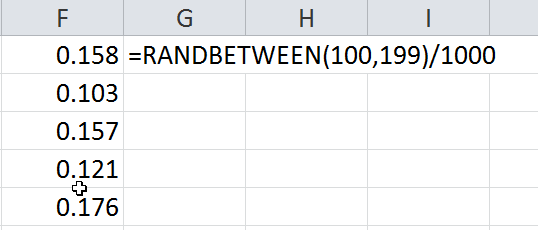Generating Text:

Tip # 03:

If you are looking texts, you can always combine the random function with the text preceded in the following manner:

Text_RANDBETWEEN(start_num,end_num)

You can repeat the step to create combinations of different fields.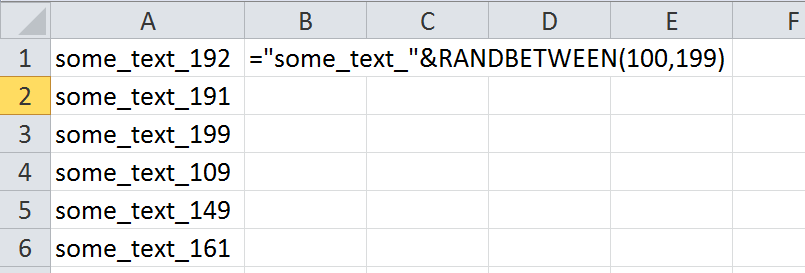Tip#04

In order to generate email ids, you can list the desired domains in a spread sheets and then choose them randomly to get the desired email id. For example if you developed the first part of email id using Text_RANDBETWEEN(start_num,end_num) with INDEX() function to add the domain randomly, later you can use concatenate to connect it to an id: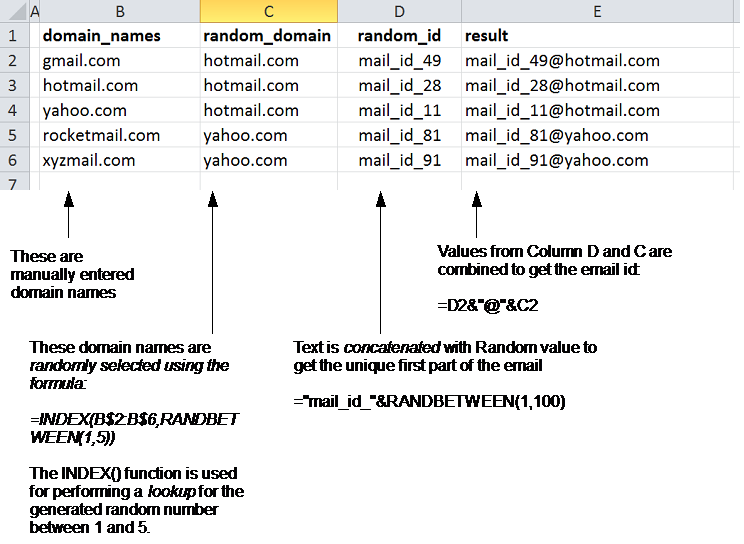Generating special formatted numbers:

Tip # 05

If you want to create credit card numbers or similar data, you can also produce it using RANDBETWEEN() formula. For example number XXX-XXXX-XX can be created by starting from 111111111 to 99999999 and formatting the number to custom format ###-####-##. See following example: